Welcome to FunTrivia's Question & Answer forum!

Search All Questions

Please cite any factual claims with citation links or references from authoritative sources. Editors continuously recheck submissions and claims.

Archived Questions

Goto Qn #

# What is the difference between a golden triangle and a golden gnomon?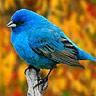star_gazer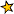star_gazer21 year member
5236 repliesThe golden triangle is uniquely identified as the only triangle to have its three angles in 2:2:1 proportion.

The golden gnomon is uniquely identified as the triangle having its three angles in 1:1:3 proportion.

A golden triangle is an isosceles triangle in which the two longer sides have equal lengths and in which the ratio of this length to that of the third, smaller side is the golden ratio:

Golden triangles are found in the nets of several stellations of dodecahedrons and icosahedrons. Also, it is the shape of the triangles found in the points of pentagrams.

Closely related to the golden triangle is the golden gnomon, the obtuse isosceles triangle in which the ratio of the length of the equal (shorter) sides to the length of the third side is the reciprocal of the golden ratio. The golden gnomon is also uniquely identified as the triangle having its three angles in 1:1:3 proportion. The acute angle is 36 degrees, the same as the smaller angle of the golden triangle.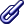https://en.wikipedia.org/wiki/Golden_triangle_(mathematics)

Mar 23 2010, 7:03 PM
navonil1
navonil1
14 year member
99 replies

The golden gnomon is the obtuse isosceles triangle whose ratio of side to base lengths is given by 1/phi=phi-1, where phi is the golden ratio. Such a triangle has angles of 36 degrees-36 degrees-108 degrees and can be constructed from a regular pentagon as illustrated above in red. The corresponding 36-72-72 triangle with side-to-base ratio phi is a golden triangle.http://mathworld.wolfram.com/GoldenGnomon.html

Mar 25 2010, 11:32 AM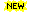FREE! Get a new mixed Fun Trivia quiz each day in your email. It's a fun way to start your day!Your Email Address:

Sign in or Create Free User ID to participate in the discussion

#### Related FunTrivia Quizzes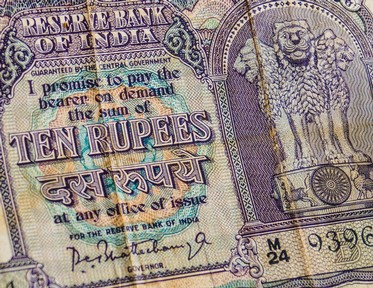India's Golden Triangle
(Individual Regions, States and Cities)The Golden World of Golden Retrievers
(Retrievers)Golden Gate, Golden State
(Bridges)